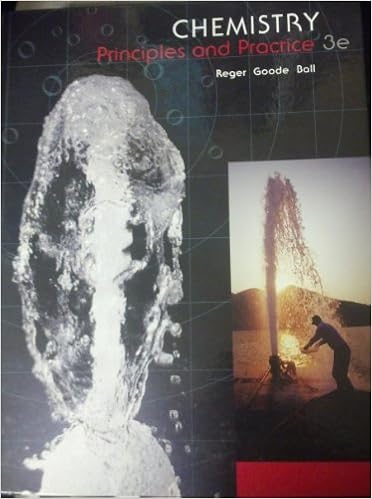# Number ü number of protons ü z number ü protons

• Notes
• 125

This preview shows page 35 - 59 out of 125 pages.

##### We have textbook solutions for you!
The document you are viewing contains questions related to this textbook.The document you are viewing contains questions related to this textbook.
Chapter 2 / Exercise 2.37
Chemistry: Principles and Practice
Reger ChemExpert Verified
__________ Number ü Number of protons ü Z _________ Number ü Protons + Neutrons ü Whole number ü A Abundance = relative amount found in a sample Isotopes
##### We have textbook solutions for you!
The document you are viewing contains questions related to this textbook.The document you are viewing contains questions related to this textbook.
Chapter 2 / Exercise 2.37
Chemistry: Principles and Practice
Reger ChemExpert Verified
How many protons, electrons, and neutrons are in a given atom ?
37 Calculating Relative Abundance =∑(fraction of isotope 1 x mass of isotope 1) + (fraction of iso 2 x mass of iso 2) + (fraction of iso 3 x mass of iso 3) + …
38
39 Isotope: form of element w/ different # of neutrons Ion : form w/ different # of electrons & protons when atoms gain or lose electrons, they acquire a charge charged particles are called _____ when atoms gain electrons, they become negatively charged ions, called _______ when atoms lose electrons, they become positively charged ions, called _______
40 The MOLE The amount of material containing 6.0221421 x 1023 particles. Avogadro’s number (NA) The numerical value of the mole is defined as being equal to the # of atoms in exactly 12 g of pure C-12 (12g of C = 1 mol C atoms = 6.022 x 1023 C atoms). 1 atom of C-12 weighs exactly 12 amu 1 mole of C-12 weighs exactly 12 g
41
42 Which weighs more: A mole of Al, C, or He?
Relationship Between Moles and Mass The mass of one mole of atoms is called the ___________
43
44
45
46
47
48 Periodic Pattern
49 Metals solids at room temperature, except Hg reflective surface shiny conduct heat conduct electricity malleable can be shaped ductile drawn or pulled into wires lose electrons and form cations in reactions about 75% of the elements are metals lower left on the table
50 Nonmetals found in all 3 states poor conductors of heat poor conductors of electricity solids are brittle gain electrons in reactions to become anions upper right on the table except H Sulfur, S( s ) Bromine, Br2( l ) Chlorine, Cl2( l )
51 Metalloids show some properties of metals and some of nonmetals also known as semiconductors Properties of Silicon shiny conducts electricity does not conduct heat well brittle
52 The Modern Periodic Table Groups or Families (__________) Elements w/ similar properties are in the same colums designated by a number and letter at top Periods (________) Each period shows the pattern of properties repeated in the next period
53
Patterns in Metallic Character = Metal = Metalloid = Nonmetal
•••